Home > mini projects > FREQUENCY SHIFT KEYING USING 555

FREQUENCY SHIFT KEYING

Abstract -Frequency shift keying modulation technique is proposed here .Communication is integral part of human being. But as communication distance increases, the strength and power of the signal decreases. Hence ,in order to better long distance communication ,we are basically used modulation techniques. Frequency shift keying ,is one of the digital modulation technique

. it have better noise immunity .the modulation stage consists of ic 555 timer working in astable mode and generating frequency shift keying (FSK)signal.

I. I NTRODUCTION

As we know communication is the basic part of our life. Communication can be analog or digital. Now -a-days communication is mostly based on digital domain.In real, digital communication is very important aspect as it is mostly used in computers and many electronics communication.

For digital data transmission, there are different modulation techniques such amplitude shift keying, frequency shift keying and phase shift keying, to achieve a better signal at reception. Frequency-shift keying (FSK) is a method of transmitting digital signals. The two binary states, logic 0 (low) and 1 (high), are each represented by an different frequency of pulse. Logic 0 is represented by a wave at a specific frequency, and logic 1 is represented by a wave at a different frequency.

Basically, it is used in modem, point to point long distance communication. A modem a device which converts the binary data from a computer to FSK for transmission over telephone lines, cables, optical fiber, or wireless media. The modem also converts incoming FSK signals to digital low and high states (ie., logic 0 and logic 1), the language into which the computer can "understand". Today, a standard telephone modem operates at thousands of bits per second. Cable and wireless modems work at more than 1,000,000 bps (one megabit per second or 1 Mbps), and optical fiber modems function at many Mbps.

II. FSK MODUALTION

A frequency shift keyed transmitter has its frequency shifted by the message. There are different types of FSK corresponding to the number of frequencies transmitted. For example in QFSK we transmit four different frequencies which corresponds to the two data bits. Although there could be more than two frequencies involved in an FSK signal, in this experiment the message will be a binary bit stream, and so only two frequencies will be involved.Figure1: FSK TRASMITTER

Its working is basically similar to a multiplexer. When logic 1 is to be transmitted it switches to one frequency(consider it to be f1) and when logic 0 is to transmitted it switches to some other frequency(consider it to be f2).In this experiment we are changing the frequency using 555 timer ic. Ic 555 is used in astable mode.

The frequency corresponds to logic'0' is called as space frequency and frequency corresponds to logic'1' is

called to mark frequency. Logic 1 frequency is low when compared to logic 0 frequncy. Just as given in figure below,

Figure2: INPUT AND OUTPUT WAVEFROMS The ic 555 pin description is given as following:

Input :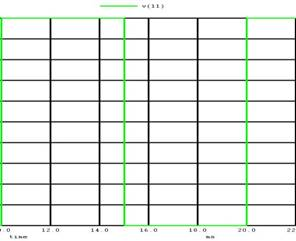Output: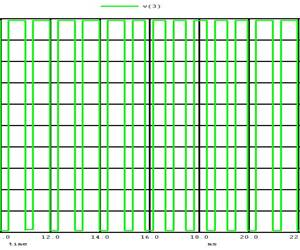III. TIMER IC

IC 555 TIMER PIN DESCRIPTION

 Pin description function 1 Ground All voltages in circuit is measured with respect to this point 2 Trigger input Output of the timer totally depends upon the amplitude of the external trigger voltage applied to this pin. 3 Output The voltage is measured with respect to ground 4 Reset Separate terminal is provided to reset flip flop externally 5 control voltage By imposing a voltage to this the width of pulse can be changed 6 Threshold voltage The timing (OUT high) interval ends when the voltage at THR (THR is greater than that at CTRL (2/3 VCC if CTRL is open). 7 Discharge Open collector output 8 vcc Supply voltage must be applied in between +5 to +18 volt

The 555 timer IC is has many applications. It is mainly used in a variety of timers, pulse generation, and oscillator applications.Ic 555 is timer can be used as monostable, astable and bistable mode of operation.

Ic 555 is used in fsk generator. Basically, timer ic used in astable mode . An Astable Multivibrator is an oscillator circuit which continuously produces rectangular wave without using an external triggering. So Astable Multivibrator is also known as Free Running Multivibrator. In monostable and bistable type of multivibrator we need an external triggering Astable Multivibrator using 555 Timer is very simple circuit, it can be implemented easily, very stable and low cost. Due to these reasons 555 has a large number of applications and it is a popular IC.

Working of astable mode:

In astable multivibrator the output changes according to the charging and discharging of the capacitor (which is at trigger pin).

Initially,consider that flip flop is set

.therefore,Q'=0.this turns OFF the transistor Q1 and output of timer is high .since,Q1 is OFF ,capacitor C starts charging up towards +vcc though resistance Ra andRb .

Capacitor is connected to threshold terminal and reference voltage of comparator-1 is 2/3 vcc,when voltage is equal to or greater than 2/3 vcc,the output of comparator1 goes high.this turns on transistor Q1 and output of timer goes low.

Circuit diagram: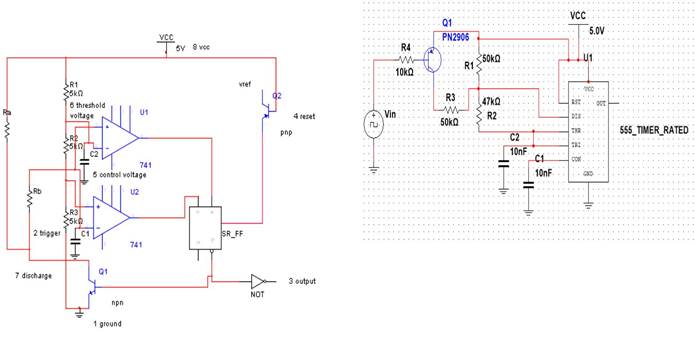FIGURE 3: 555 TIMER IC IN ASTABLE MODE

Now,capacitor C discharges through transistor Q1 and resistor rb.when capacitor falls below 1/3 vcc ,which is reference

voltage of comparator 2 ,the output of comparator goes highand flip flop sets. hence Q'=0 .again transistor Q1 turns

OFF and output of timer is high. Again, process is repeated.

Capacitor charging time,tc=0.69(Ra+Rb)C

Capacitor discharging time,td=0.69RbC

Total time period of waveform=T=0.69(Ra+2*Rb)*c

Frequency ,f=1.45/(Ra+2*Rb)*C

It consists of a IC 555Timer which works in Astable Mode. Here ,transistor pnp act as a switch.The resistors R1 and R2and capacitor C determines the Frequency of the FSK modulated signal. The standard digital data input frequency is usually 150Hz. When the input is HIGH i.e. when the input binary data is of logic 1, the PNP transistor Q is off and IC 555 Timer works in the astable mode of operation. The frequency of the output FSK modulated signal is given by the Equation.

 f= 1.45/(R1+2R2)C2 (1)

The circuit diagram when the input is HIGH is shown in the Figure 5.FIGURE 4: FSK MODULATION USING 555 TIME IC

IV. MODULATION

 Modulation is the process of altering the Characteristics or changing the parameters of the carrier signal in accordance to the message signal. Here Frequency Shift Keying Modulated signal is obtained using IC 555 Timer.frequency shift keying is modulation in which it changes carrier

frequency according to logic levels. The

circuit diagram for FSK Signal generation using IC

555 Timer is shown in Figure 4. Here the values of R1= 50K ohm, R2= 47K ohm

and C=10nF. When the input is LOW i.e. when the

input binary data is of logic 0, the PNP transistor Q is on and its connects the resistance R3 , across the resistance R1. Thus now the frequency of the output FSK modulated signal is given by the equation

 f= 1.45/((R1||R3)+2R2)C2 (2)

The circuit diagram when the

input is LOW is shown in the Figure 6.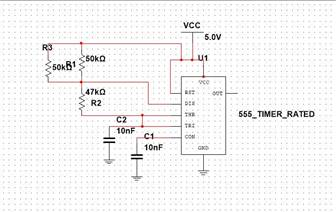Here,

the value of R3=50K ohm. The capacitor C1 is used to

bypass noise and ripples from the supply. Mark frequency and space frequency are as shown in figure 7.V. CALCULATIONS

C1=10nF

C2=10Nf

1)mark frequency

Frequency= f= 1.45/(R1+2R2)C2 =1.45/(50+2*47)*10*^(3)*10*10^(-9) =1008HZ

2)space frequency

Frequency= f= 1.45/(R1||R3+2R2)C2 =1.45/(50||50+2*47)*10*^(3)*10*10^(-9) =1218HZ

VI. WAVEFORMS

INPUT WAVEFORM

Frequency =100hz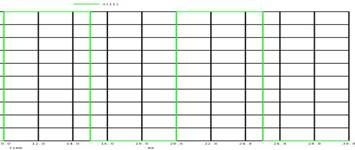OUTPUT WAVEFORM

Space frequency=1218HZ

Mark frequency=1008HZBoth plot of input and output waveform: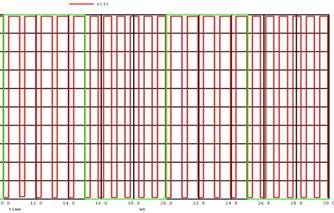The major disadvantage is its high bandwidth requirement compared to ASK. Therefore FSK is extensively used in low speed modems having bit rates below 1200 bits/sec. The FSK is not preferred for the high speed modems because as we know ,with increase in speed, the bit rate also increases.This increases the channel bandwidth required to transmit the FSK signal. As the telephone lines have a very low bandwidth, it is not possible to satisfy the bandwidth requirement of FSK at higher speed. Therefore FSK is preferred only for the low speed modems.

VIII. CONCLUSION

Specification:

 Sr.no. frequency value 1. Mark frequency 1008HZ 2. Space frequency 1218HZ

VII. APPLICATIONS

It is used Point to point military communication and telephone modems. The technology is used for